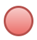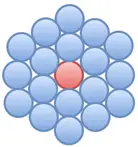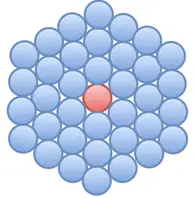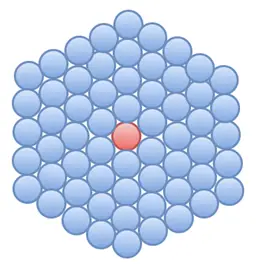# Electrical Stranded Conductors

Stranded conductors are very much popular in electrical power system for transmission and distribution line. A stranded conductor is consists of several thin wires of small cross sectional area called strands as shown in figure below-As shown in figure above, at the center of stranded conductor, we are using steel conductor which provided the high tensile strength to conductor. In the outer layers of stranded conductor, we use aluminum conductors, which provide the conductivity to stranded conductor.

Basic, reason of using stranded conductor is to make the conductor flexible. If we use a single solid conductor. It does not have sufficient flexibility and it is difficult to coil a solid conductor. Hence, it becomes difficult to transport a single solid conductor of long length over the distance. To eliminate this drawback, conductor is formed by using several thin wires of small cross section. These thin wires are called strands. By making the conductor stranded, it becomes flexible. Which makes stranded conductor suitable to be coiled easily to transport it over long distance.

There are some facts to be noted about stranded conductors-

1. The stranded conductor is having sufficient flexibility, which makes stranded conductor suitable to be coiled easily to transport it over long distance.
2. For a stranded conductor of same cross sectional area, the flexibility of conductor increase with increase of number of strands in conductor.
3. The stranded conductor is formed by twisting the strands together in layers.
4. The strands of each layer are laid in helical fashion over the preceding layer. This process is called stranding.
5. Generally, in successive layer, the stranding is done in opposite direction to preceding layer. This mean, if the strands of one layer are twisted in clockwise direction, the strands of next layer will be twisted in anticlockwise direction and so on ‘x’ is number of layers in conductor.
6. Generally, the total number of strands in any conductor is given by the formulae of,Where, N is total number of strands in stranded conductor.
7. Generally the diameter of conductor can be calculated by using the formula of,Where, D is the diameter of conductor,
‘d’ is the diameter of each strand.

Table Representing the Number of Strands, Diameter and Cross-Sectional view of Stranded Conductor for Different no. of Layers

 Sl No. No. of layers ‘x’ Total no. of strands N = 3x2 – 3x + 1 Diameter of conductor D = (2x – 1)d Cross Sectional View of Stranded Conductor 1 1 1 d2 2 7 3d3 3 19 5d4 4 37 7d5 5 61 9dWant More Electrical Knowledge?
Enter your email below to receive FREE informative articles on Electrical & Electronics Engineering